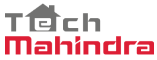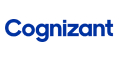New update is available. Click here to update.
Topics

# Armstrong Number

Easy0/40
Average time to solve is 15m+13 more companies

## Problem statement

You are given an integer ‘NUM’ . Your task is to find out whether this number is an Armstrong number or not.

A k-digit number ‘NUM’ is an Armstrong number if and only if the k-th power of each digit sums to ‘NUM’.

Example
``````153 = 1^3 + 5^3 + 3^3.

Therefore 153 is an Armstrong number.
``````
Detailed explanation ( Input/output format, Notes, Images )
Constraints:
``````1 <= ‘T’ <= 100
1 <= ‘N’ <= 10^9

Time Limit: 1 sec
``````
Sample Input 1:
``````1
13
``````
Sample Output 1:
``````NO
``````
Explanation For Sample Input 1:
``````As 1^2 + 3^2 = 10 which is not equal to 13.So we can say it is not an Armstrong number.
``````
Sample Input 2:
``````1
371
``````
Sample Output 2:
``````YES
``````Console